## 2. Intersecting Curves

### a. Street Lines

 Coordinates of point A are 2000.00' N and 500.00' E. Using the curve geometry shown, compute coordinates of the labeled points.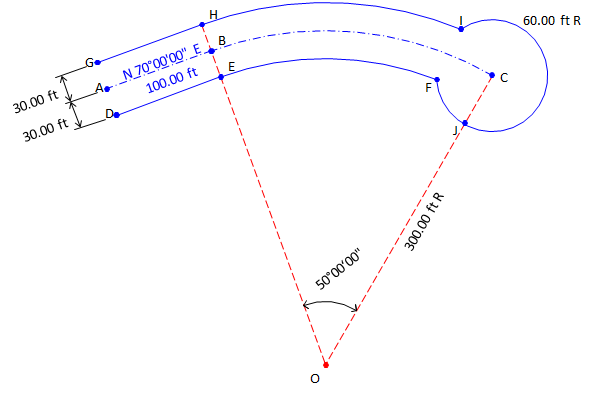Because sine and cosine of Azimuths will always return the correct mathematical sign in coordinate computations, they will be used instead of Bearings. Starting at point A, compute azimuths to the side points and all the way to point CSide points G and D at A.Side points H and E at B; Continue to center of arc then cul-de-sac center.Compute sideline points D and G coordinates from point A:Compute point B from point A:Then the sideline points H and E from B: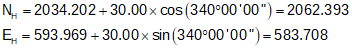Starting at point B, compute points O, J, and C: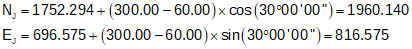Point F cannot be directly computed from a known point. Because its position is defined by the intersection of the southerly street sideline and the cul-de-sac, it is connected by radii to points O and C. Its position is computed from a distance-distance intersectionWe have the length and direction of the baseline OC. We also have lengths of the other two sides of the intersection triangle: dCF = 60.00' and  dOF = 300.00'-30.00' = 270.00' Because a distance-distance intersection results in two possible point locations, we must take care to solve the correct triangle.Use Law of Cosines to solve angle at C:Then the direction from C to F:And finally the coordinates from point C:Similarly, point I is at the intersection of the northerly street sideline and the cul-de-sac. Intersection traingle sides are 300.00', 60.00' and 330.00'. As before, there are two possible intersection locations. After determining correct triangle to solve: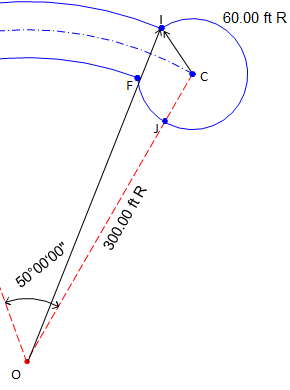Use Law of Cosines to solve angle at C: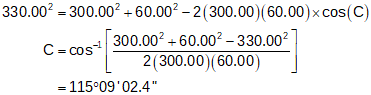Then the direction from C to I:And finally the coordinates from point C:Coordinates Summary:### b. Curvilinear Traverse

Lets add a lot and compute its coordinates and area.Starting at point C, compute points Q, R, and S. Convert bearings to azimuhts for ease of computations.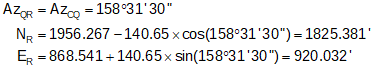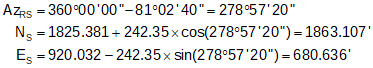Point T must be computed by intersecting the westerly lot line with the southerly street sideline. Point T is connected to point S by a bearing and point O by the southerly curve radius.Line SO will serve as the baseline for the intersection triangle. Compute its length and direction from point S's and O's coordinates.Compute angle at S from directions of SO and ST: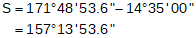Use Law of Sines to solve angle at T:(since the angle at T is less than 90°, this is the correct angle). Compute angle at O:And length of line ST:Finally, compute point T from point S:To determine the Lot area, we will use the coordinate method then take into account the segment areas caused by the two arcs. To compute the segment areas we need the central angle for each arc.

 The following equations come from the VII COGO topic. Segment area:(Eqn F-7) Central angle: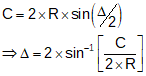(Eqn F-3)

Coordinate method area:Arc TF:Arc FQTotal Lot area: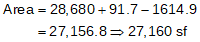Final Lot dimensions: